# Previous year Questions (2016-20) - Inverse Trigonometric Functions Notes | EduRev

## JEE : Previous year Questions (2016-20) - Inverse Trigonometric Functions Notes | EduRev

The document Previous year Questions (2016-20) - Inverse Trigonometric Functions Notes | EduRev is a part of the JEE Course Mathematics (Maths) Class 12.
All you need of JEE at this link: JEE

Q.1.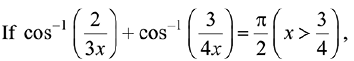then x is equal to:    (2019)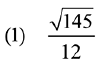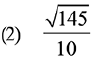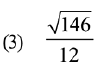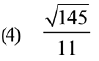Ans. (1)
Solution.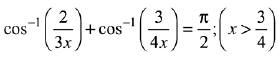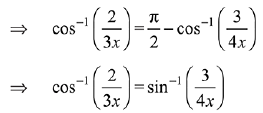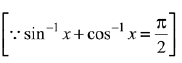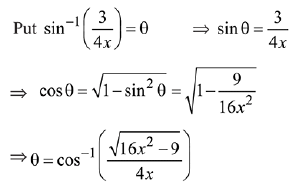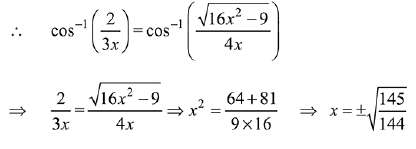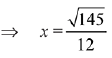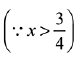Q.2. If x = sin-1 (sin 10) and y = cos-1 (cos 10), then y - x is equal to:   (2019)
(1) 0
(2) 10
(3) 7 π
(4) π

Ans. (4)
Solution.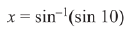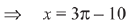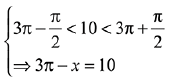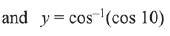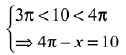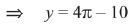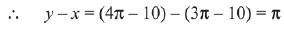Q.3.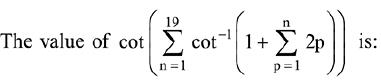(2019)
(1) 21/19
(2) 19/21
(3) 22/23
(4) 23/22
Ans. (1)
Solution.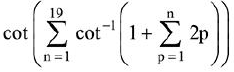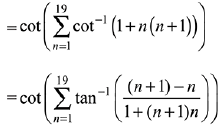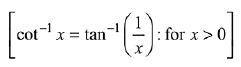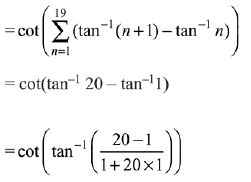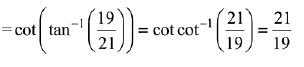Q.4. All x-satisfying the inequality (cot-1 x)- 7(cot-1x)+ 10 > 0, lie in the interval:    (2019)
(1) (-∞, cot 5) ∪ (cot 4, cot 2)
(2) (cot 2, ∞)
(3) (-∞, cot 5) ∪ (cot 2, ∞)
(4) (cot 5, cot 4)

Ans. (2)
Solution.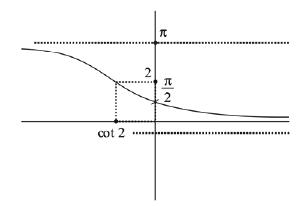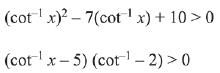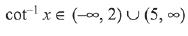...(1)
But cot-1 x lies in (0, π)
Now, from equation (1)
cot-1x ∈  (0, 2)
Now, it is clear from the graph
x ∈ (cot 2, ∞)

Q.5. Considering only the principal values of inverse functions, the set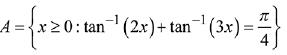(2019)
(1) contains two elements
(2) contains more than two elements
(3)  is a singleton
(4) is an empty set

Ans. (3)
Solution.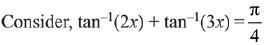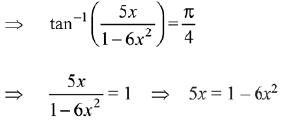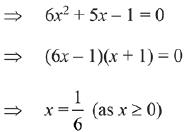Therefore, A is a singleton set.

Q.6.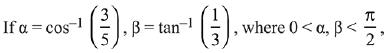then α - β is equal to:    (2019)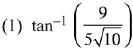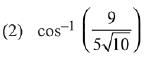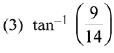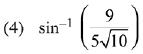Ans. (4)
Solution.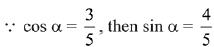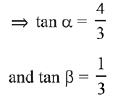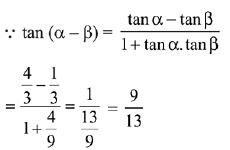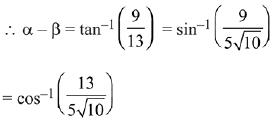Q.7.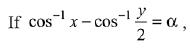where -1 ≤ x ≤ 1, -2 ≤ y ≤ 2,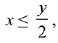then for all x, y, 4x2 -4xy cosα +y2 is equal to:    (2019)
(1) 4 sin2α
(2) 2 sin2α
(3) 4 sin2α - 2x2y2
(d) 4 cos2 α + 2x2y2

Ans. (1)
Solution.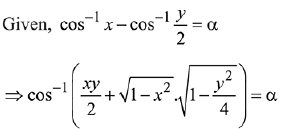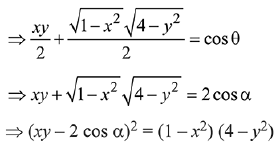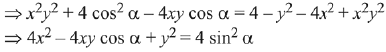Q.8. The value of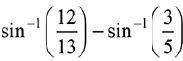is equal to:    (2019)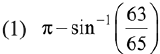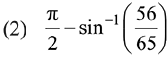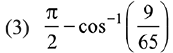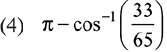Ans. (2)
Solution.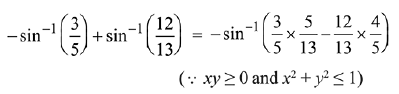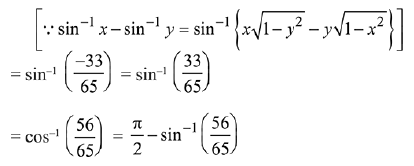Q.9. If for x∈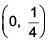the derivative of tan-1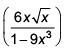is √x × g(x) , then g(x) equals     (2017)
(1)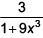(2)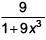(3)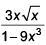(4)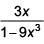Ans.
(2)
Solution.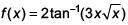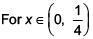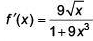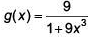Q.10. The value of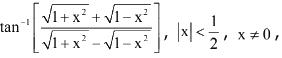is equal to:     (2017)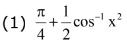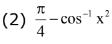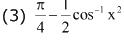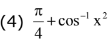Ans. (1)
Solution.
x2 = cos2θ ; θ =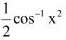=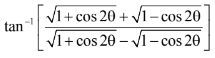=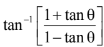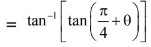=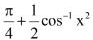Q.11. A value of x satisfying the equation sin[cot-1(1+x)]=cos[tan-1x], is:    (2017)
(1)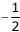(2) 0
(3) -1
(4) 1/2
Ans. (1)
Solution.
Given that sin[cot-1 (x+1)] = cos (tan-1x) ......(i)
We know that,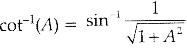Here, A = x + 1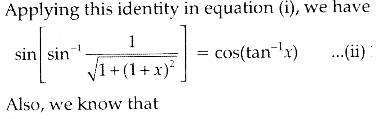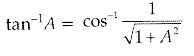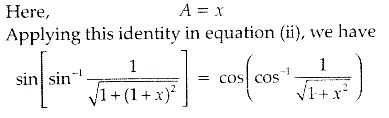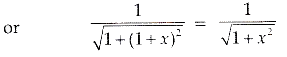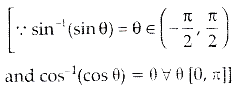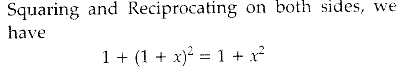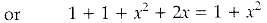or 1 + 2x = 0
or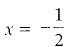Q.12. Consider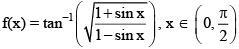A normal to y = f(x) at x = π/6 also passes through the point:    (2016)
(1) (0, 0)
(2) (0, 2π/3)
(3) (π/6, 0)
(4) (π/4, 0)
Ans.
(2)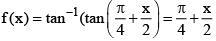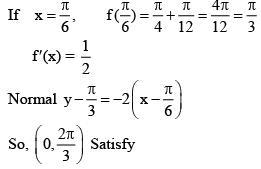Offer running on EduRev: Apply code STAYHOME200 to get INR 200 off on our premium plan EduRev Infinity!

## Mathematics (Maths) Class 12

209 videos|222 docs|124 tests

,

,

,

,

,

,

,

,

,

,

,

,

,

,

,

,

,

,

,

,

,

;